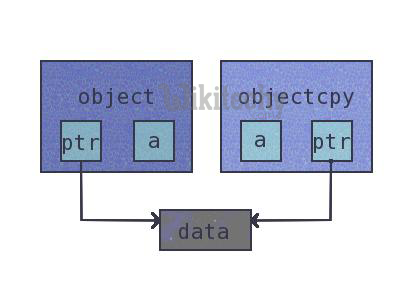# python tutorial - Advanced python interview questions - learn python - python programming

## What Is The Command To Debug A Python Program?

• The following command helps run a Python program in debug mode.

## How Do You Monitor The Code Flow Of A Program In Python?

• In Python, we can use <sys> module’s <settrace()> method to setup trace hooks and monitor the functions inside a program.Learn python - python tutorial - python flow charts - python examples - python programs

• You need to define a trace callback method and pass it to the <settrace()> method. The callback should specify three arguments as shown below.

## Why And When Do You Use Generators In Python?

• A generator in Python is a function which returns an iterable object. We can iterate on the generator object using the <yield> keyword. But we can only do that once because their values don’t persist in memory, they get the values on the fly.
• Generators give us the ability to hold the execution of a function or a step as long as we want to keep it. However, here are a few examples where it is beneficial to use generators.
• We can replace loops with generators for efficiently calculating results involving large data sets.
• Generators are useful when we don’t want all the results and wish to hold back for some time.
• Instead of using a callback function, we can replace it with a generator. We can write a loop inside the function doing the same thing as the callback and turns it into a generator.

## What Does The <Yield> Keyword Do In Python?

• The <yield> keyword can turn any function into a generator. It works like a standard return keyword.
• But it’ll always return a generator object. Also, a function can have multiple calls to the <yield> keyword.

## How To Convert A List Into Other Data Types?

• Sometimes, we don’t use lists as is. Instead, we have to convert them to other types.

## Turn A List Into A String.

• We can use the <”.join()> method which combines all elements into one and returns as a string.

## Turn A List Into A Tuple.

• Call Python’s <tuple()> function for converting a list into a tuple. This function takes the list as its argument. But remember, we can’t change the list after turning it into a tuple because it becomes immutable.

## Turn A List Into A Set.

• Converting a list to a set poses two side-effects.
• Set doesn’t allow duplicate entries, so the conversion will remove any such item if found.
• A set is an ordered collection, so the order of list items would also change.
• However, we can use the <set()> function to convert a list to a set.

## Turn A List Into A Dictionary.

• In a dictionary, each item represents a key-value pair. So converting a list isn’t as straight forward as it were for other data types.
• Passing the tuples into the <dict()> function would finally turn them into a dictionary.

## How Do You Count The Occurrences Of Each Item Present In The List Without Explicitly Mentioning Them?

• Unlike sets, lists can have items with same values. In Python, the list has a <count()> function which returns the occurrences of a particular item.
• Count The Occurrences Of An Individual Item.

## output:

• Count The Occurrences Of Each Item In The List.
• We’ll use the list comprehension along with the <count()> method. It’ll print the frequency of each of the items.

## What Is NumPy And How Is It Better Than A List In Python?

• NumPy arrays are more compact than lists.
• Reading and writing items is faster with NumPy.
• Using NumPy is more convenient than to the standard list.
• NumPy arrays are more efficient as they augment the functionality of lists in Python.

## What Are Different Ways To Create An Empty NumPy Array In Python?

• There are two methods which we can apply to create empty NumPy arrays.
• The First Method To Create An Empty Array.
• The Second Method To Create An Empty Array.

## How can you copy objects in Python?

• The functions used to copy objects in Python are
• Copy.copy () for shallow copy
• Copy.deepcopy () for deep copyLearn python - python tutorial - python copy - python examples - python programs

## What is the difference between tuples and lists in Python?

• Tuples can be used as keys for dictionaries i.e. they can be hashed. Lists are mutable whereas tuples are immutable - they cannot be changed.
• Tuples should be used when the order of elements in a sequence matters. For example, set of actions that need to be executed in sequence, geographic locations or list of points on a specific route.